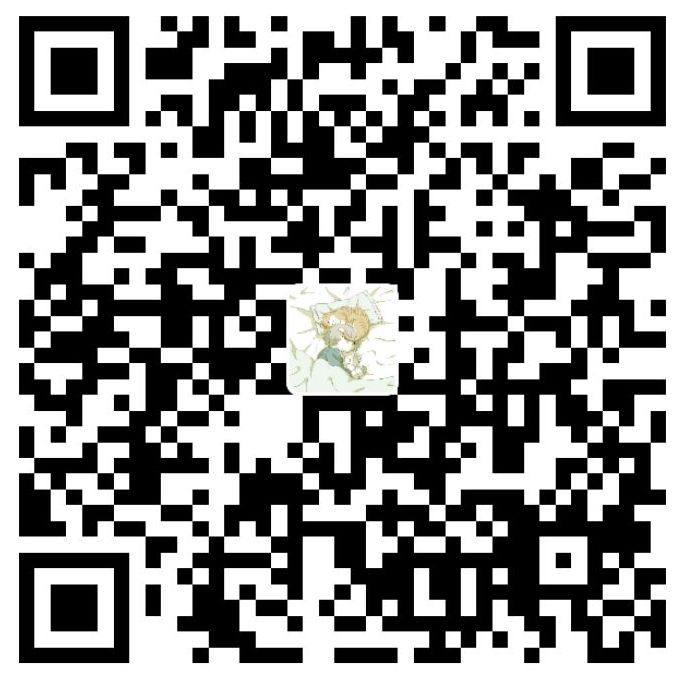### 一、前言

• 没有足够的交互来为模型学习提供信息。此外，数据高度不平衡：大多数用户和物品与系统只有很少的交互，这使得推荐任务更加困难。
• 由于我们在隐式反馈中只观察到一小部分正样本，现有的负采样策略是将未观察到的样本视为负样本。然而，通过这种方式，许多潜在的正样本被错误地标记为负样本，并且模型被标签噪声严重误导，尤其是在稀疏数据上。

### 二、Text Memory Network(TMN)

$S$ 是word2vec在GoogleNews语料库上预训练的词语义矩阵， $S_w$ 表示词 $w$ 的语义特征。我们使用 $E$ 和 $F$ 分别表示我们为用户和物品构建的文本特征。以用户 $u$ 为例，$E_u=\mathop{\sum}\limits_{w\in R_u}a_{uw}S_w$ ，其中 $a_{uw}$ 是词 $w$ 基于 $u$ 的语义偏好的权重。我们提出了一个文本记忆网络 (TMN) 来计算用户 $a_{uw}$ 和物品 $a_{iv}$ 的权重，以根据单词语义构建文本特征。

$$L=-\mathop{\sum}\limits_{u,i}R_{ui}log\hat{R}_{ui}+(1-R_{ui})log(1-\hat{R}_{ui})$$

$$\hat{R}_{ui}=\sigma[(\mathop{\sum}\limits_{w\in R_u}\frac{exp(P_uT_w^T)}{\mathop{\sum}\limits_{w'\in R_u}exp(P_uT_{w'}^T)})(\mathop{\sum}\limits_{v\in R_i}\frac{exp(Q_iT_v^T)}{\mathop{\sum}\limits_{v'\in R_i}exp(Q_iT_{v'}^T)})]$$

### 三、文本增强跨域推荐

#### 3.1 文本协同过滤TCF

$$\hat{R}_{ui}=f([U,E]_u,[V,F]_i,\Theta)$$

#### 3.2 文本增强域自适应推荐

$$\hat{R}_{u^si^s}^s=f([U^s,E^s]_{u^s},[V^s,F^s]_{i^s},\Theta)$$

$$\hat{R}_{u^ti^t}^t=f([U^t,E^t]_{u^t},[V^t,F^t]_{i^t},\Theta)$$### 四、参考链接微信支付宝